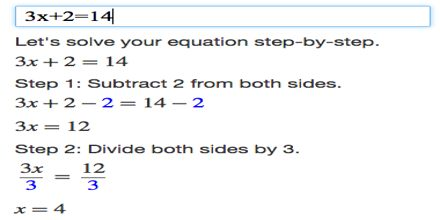Mathematic

# Algebra TutorialGeneral objective of this lecture is to present on Algebra Tutorial. Algebra the part of mathematics in which letters and other general symbols are used to represent numbers and quantities in formulae and equations. In elementary algebra, those symbols represent quantities without fixed values, known as variables. Just as sentences describe relationships between specific words, in algebra, equations describe relationships between variables.